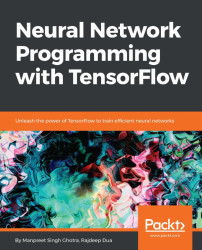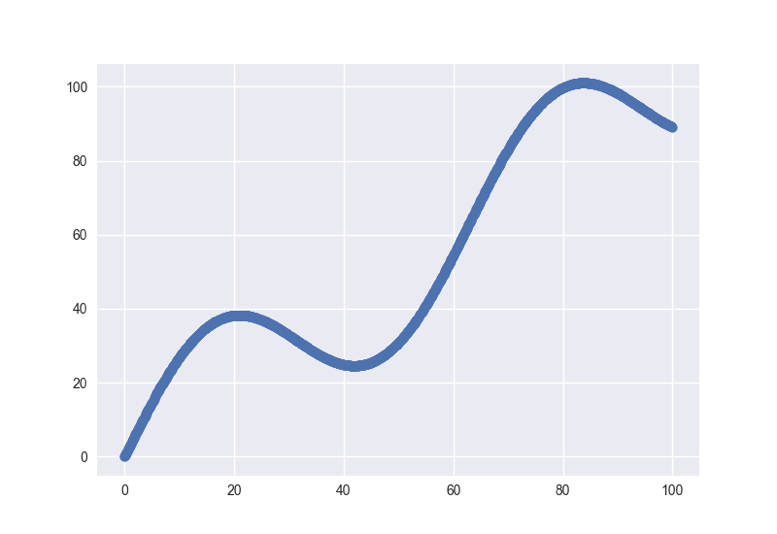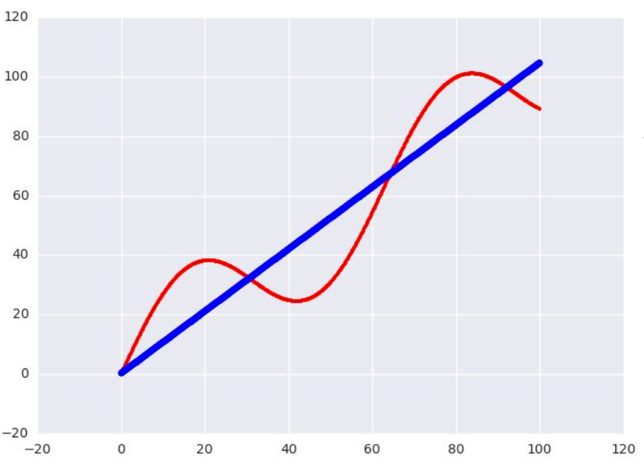•#### Neural Network Programming with Tensorflow#### Overview of this book

If you're aware of the buzz surrounding the terms such as "machine learning," "artificial intelligence," or "deep learning," you might know what neural networks are. Ever wondered how they help in solving complex computational problem efficiently, or how to train efficient neural networks? This book will teach you just that. You will start by getting a quick overview of the popular TensorFlow library and how it is used to train different neural networks. You will get a thorough understanding of the fundamentals and basic math for neural networks and why TensorFlow is a popular choice Then, you will proceed to implement a simple feed forward neural network. Next you will master optimization techniques and algorithms for neural networks using TensorFlow. Further, you will learn to implement some more complex types of neural networks such as convolutional neural networks, recurrent neural networks, and Deep Belief Networks. In the course of the book, you will be working on real-world datasets to get a hands-on understanding of neural network programming. You will also get to train generative models and will learn the applications of autoencoders. By the end of this book, you will have a fair understanding of how you can leverage the power of TensorFlow to train neural networks of varying complexities, without any hassle. While you are learning about various neural network implementations you will learn the underlying mathematics and linear algebra and how they map to the appropriate TensorFlow constructs.
Title PageCreditswww.PacktPub.comCustomer FeedbackPrefaceFree Chapter
Maths for Neural NetworksDeep Feedforward NetworksOptimization for Neural NetworksConvolutional Neural NetworksRecurrent Neural NetworksGenerative ModelsDeep Belief NetworkingAutoencodersResearch in Neural NetworksGetting started with TensorFlow## Optimization

As part of deep learning, we mostly would like to optimize the value of a function that either minimizes or maximizes f(x) with respect to x. A few examples of optimization problems are least-squares, logistic regression, and support vector machines. Many of these techniques will get examined in detail in later chapters.

### Optimizers

We will study `AdamOptimizer` here; TensorFlow `AdamOptimizer` uses Kingma and Ba's Adam algorithm to manage the learning rate. Adam has many advantages over the simple `GradientDescentOptimizer`. The first is that it uses moving averages of the parameters, which enables Adam to use a larger step size, and it will converge to this step size without any fine-tuning.

The disadvantage of Adam is that it requires more computation to be performed for each parameter in each training step. `GradientDescentOptimizer` can be used as well, but it would require more hyperparameter tuning before it would converge as quickly. The following example shows how to use `AdamOptimizer`:

• `tf.train.Optimizer` creates an optimizer
• `tf.train.Optimizer.minimize(loss, var_list)` adds the optimization operation to the computation graph

Here, automatic differentiation computes gradients without user input:

```import numpy as np
import seaborn
import matplotlib.pyplot as plt
import tensorflow as tf

# input dataset
xData = np.arange(100, step=.1)
yData = xData + 20 * np.sin(xData/10)

# scatter plot for input data
plt.scatter(xData, yData)
plt.show()

# defining data size and batch size
nSamples = 1000
batchSize = 100

# resize
xData = np.reshape(xData, (nSamples,1))
yData = np.reshape(yData, (nSamples,1))

# input placeholders
x = tf.placeholder(tf.float32, shape=(batchSize, 1))
y = tf.placeholder(tf.float32, shape=(batchSize, 1))

# init weight and bias
with tf.variable_scope("linearRegression"):
W = tf.get_variable("weights", (1, 1), initializer=tf.random_normal_initializer())
b = tf.get_variable("bias", (1,), initializer=tf.constant_initializer(0.0))

y_pred = tf.matmul(x, W) + b
loss = tf.reduce_sum((y - y_pred)**2/nSamples)

# optimizer
with tf.Session() as sess:
sess.run(tf.global_variables_initializer())

# gradient descent loop for 500 steps
for _ in range(500):
# random minibatch
indices = np.random.choice(nSamples, batchSize)

X_batch, y_batch = xData[indices], yData[indices]